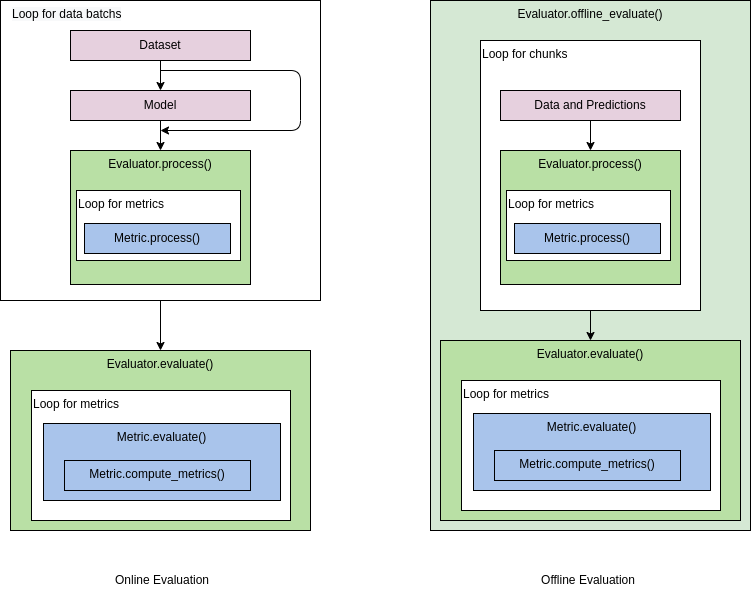Shortcuts

# Evaluation¶

## Evaluation metrics and evaluators¶

In model validation and model testing, it is often necessary to quantitatively evaluate the model’s performance. In MMEngine, Metric and Evaluator are implemented to achieve this function.

• Metric calculates specific model metrics based on test data and model prediction results. Common metrics for corresponding tasks are provided in each OpenMMLab algorithm library, e.g. Accuracy is provided in MMPreTrain for calculating the Top-k classification accuracy of classification models; COCOMetric is provided in MMDetection to calculate AP, AR, and other metrics for object detection models. The evaluation metrics are decoupled from the dataset, such as COCOMetric can also be used on non-COCO object detection datasets.

• Evaluator is an upper-level module for Metric, usually containing one or more metrics. The role of the evaluator is to perform necessary data format conversions during model evaluation and call evaluation metrics to calculate model accuracy. Evaluator is usually built from Runner or test scripts for online and offline evaluations, respectively.

### BaseMetric¶

BaseMetric is an abstract class with the following initialization parameters:

• collect_device: device name used for synchronizing results in distributed evaluation, such as 'cpu' or 'gpu'.

• prefix: the prefix of the metric name which is used to distinguish multiple metrics with the same name. If this parameter is not given, then an attempt is made to use the class attribute default_prefix as the prefix.

class BaseMetric(metaclass=ABCMeta):

default_prefix: Optional[str] = None

def __init__(self,
collect_device: str = 'cpu',
prefix: Optional[str] = None) -> None:
...


BaseMetric has the following two important methods that need to be overridden in subclasses:

• process() is used to process the test data and model prediction results for each batch. The processing results should be stored in the self.results list, which will be used to calculate the metrics after processing all test data. This method has the following two parameters:

• data_batch: A sample of test data from a batch, usually directly from the dataloader

• data_samples: Corresponding model prediction results. This method has no return value. The function interface is defined as follows:

@abstractmethod
def process(self, data_batch: Any, data_samples: Sequence[dict]) -> None:
"""Process one batch of data samples and predictions. The processed
results should be stored in self.results, which will be used to
compute the metrics when all batches have been processed.
Args:
data_batch (Any): A batch of data from the dataloader.
data_samples (Sequence[dict]): A batch of outputs from the model.
"""

• compute_metrics() is used to calculate the metrics and return the metrics in a dictionary. This method has one parameter:

• results: list type, which holds the results of all batches of test data processed by the process() method. This method returns a dictionary that holds the names of the metrics and the corresponding values of the metrics. The function interface is defined as follows:

@abstractmethod
def compute_metrics(self, results: list) -> dict:
"""Compute the metrics from processed results.

Args:
results (list): The processed results of each batch.

Returns:
dict: The computed metrics. The keys are the names of the metrics,
and the values are corresponding results.
"""


In this case, compute_metrics() is called in the evaluate() method; the latter collects and aggregates intermediate processing results of different ranks during the distributed testing before calculating the metrics.

Note that the content of self.results depends on the implementation of the subclasses. For example, when the amount of test samples or model output data is large (such as semantic segmentation, image generation, and other tasks) and it is not appropriate to store them all in memory, you can store the metrics computed by each batch in self.results and collect them in compute_metrics(); or store the intermediate results of each batch in a temporary file, and store the temporary file path in self .results, and then collect them in compute_metrics() by reading the data from the file and calculates the metrics.

## Model evaluation process¶

Usually, the process of model accuracy evaluation is shown in the figure below.

Online evaluation: The test data is usually divided into batches. Through a loop, each batch is fed into the model in turn, yielding corresponding predictions, and the test data and model predictions are passed to the evaluator. The evaluator calls the process() method of the Metric to process the data and prediction results. When the loop ends, the evaluator calls the evaluate() method of the metrics to calculate the model accuracy of the corresponding metrics.

Offline evaluation: Similar to the online evaluation process, the difference is that the pre-saved model predictions are read directly to perform the evaluation. The evaluator provides the offline_evaluate interface for calling the Metrics to calculate the model accuracy in an offline way. In order to avoid memory overflow caused by processing a large amount of data at the same time, the offline evaluation divides the test data and prediction results into chunks for processing, similar to the batches in online evaluation.## Customize evaluation metrics¶

In each algorithm library of OpenMMLab, common evaluation metrics have been implemented in the corresponding tasks. For example, COCO metrics is provided in MMDetection and Accuracy, F1Score, etc. are provided in MMPreTrain.

Users can also add custom metrics. For details, please refer to the examples given in the tutorial documentation.

© Copyright 2022, mmengine contributors. Revision 85c0976b.

Built with Sphinx using a theme provided by Read the Docs.
Versions
latest
stable
v0.10.1
v0.10.0
v0.9.1
v0.9.0
v0.8.5
v0.8.4
v0.8.3
v0.8.2
v0.8.1
v0.8.0
v0.7.4
v0.7.3
v0.7.2
v0.7.1
v0.7.0
v0.6.0
v0.5.0
v0.4.0
v0.3.0
v0.2.0